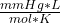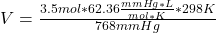## 3. What volume would be occupied by 3.5 mol of oxygen gas at a pressure of 768 mm Hg and a temperature fo 25°C? SHOWING WORK

Question

3. What volume would be occupied by 3.5 mol of oxygen gas at a pressure
of 768 mm Hg and a temperature fo 25°C?
SHOWING WORK
NO FILES PLZ

in progress 0
4 weeks 2021-08-18T04:07:16+00:00 1 Answers 0 views 0

The oxygen will occupied 84.7 L.

Explanation:

Ideal gases are a simplification of real gases that is done to study them more easily. It is considered to be formed by point particles, do not interact with each other and move randomly. It is also considered that the molecules of an ideal gas, in themselves, do not occupy any volume.

The pressure, P, the temperature, T, and the volume, V, of an ideal gas, are related by a simple formula called the ideal gas law:

P*V = n*R*T

where P is the gas pressure, V is the volume that occupies, T is its temperature, R is the ideal gas constant, and n is the number of moles of the gas. The universal constant of ideal gases R has the same value for all gaseous substances.

In this case:

• P= 768 mmHg
• V= ?
• n= 3.5 mol
• R= 62.36• T= 25 C= 298 K (being 0 C= 273 K)

Replacing:

768 mmHg* V= 3.5 mol* 62.36*298 K

Solving:V= 84.7 L

The oxygen will occupied 84.7 L.